Next: Cosmic Protons Up: Cosmic Rays Previous: Cosmic Rays   Contents

## Cosmic Electrons

Assuming that the initial spectrum for cosmic ray electrons can be represented by a power law:(1.3)

the spectrum evolution follows the law :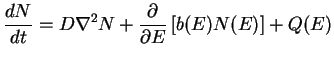(1.4)

where D is the diffusion coefficient and :(1.5)

Equation 1.4 can be resolved in first approximation by ignoring the terms due to diffusion (assuming e.g. a uniform sources distribution) and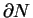/we obtain(1.6)

and integrating :(1.7)

The shape of the initial particle (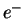) spectrum is therefore modified according to the dependence ofon. The energy loss can be due to:
• Synchrotron losses: due to the interaction of electrons with the galactic magnetic field and producing a radio emission detectable in the MHz range. This energy loss is proportional to the magnetic field energy density and to the square of the electron Lorentz factor. For this reason the effect on N(E) is especially important above a critical energy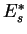, causing, for E, N(E) to become steeper and proportional to• Inverse Compton losses: they are due to the interaction with the interstellar radiation field and with the cosmic background radiation and produce emission in the gamma ray range (see par 1.5.3). As the synchrotron losses, they are proportional to the square of the electrons Lorentz factor and dominate at high energy. Also in this case the photon spectrum becomes proportional toabove a critical energy.
• Bremsstrahlung losses: they are due to interaction with the atomic nuclei of the interstellar matter and produce gamma emission (see par. 1.5.2). They are proportional to the electron energy and do not cause a change in the electron spectral shape.
• Ionization losses: they are due to interaction with interstellar matter atoms. They have a slight dependence on the electrons energy and the spectrum become proportional toThe radio observation of the synchrotron diffuse emission cover the range between few tens of MHz to a few GHz. These observations show a radiation spectrum with indexbetween 2.4 and 3, which corresponds to a spectral index of the electron flux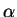between 2 and 3. In particular an increase offrom 2.4-2.6 to 2.8-3 is observed for frequency larger than 400 MHz [Strong et al., 2000]. This can be connected to the spectral break due to synchrotron and inverse Compton energy losses, as is foreseen for electron spectrum at energy of about few GeV.
The spectrum of cosmic electrons can also be obtained from the direct measurements performed at the top of the atmosphere, for energies larger than some GeV, where the solar modulation is not important. Recent measurements found that the local electron spectrum can be fitted by a single power-law, with index 3.4, up to 2-3 GeV [Casadei & Bindi, 2004].
However the electron distribution is expected to be very inhomogeneous for energies above some tens of GeV, as more energetic electrons are confined close to the sources [Strong et al., 2000], [Pohl & Esposito, 1998]. This suggests that the spectrum of cosmic electrons can be different from that measured locally.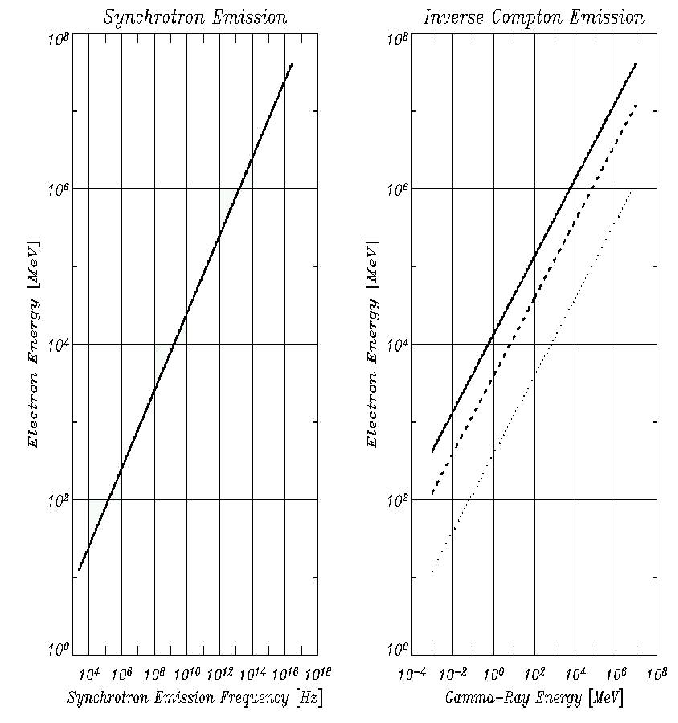Next: Cosmic Protons Up: Cosmic Rays Previous: Cosmic Rays   Contents
Andrea Giuliani 2005-01-21